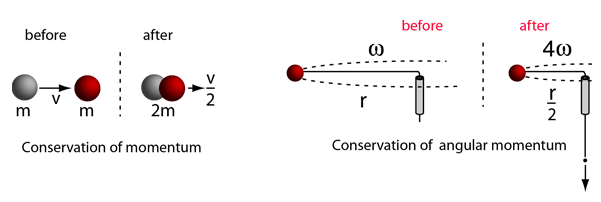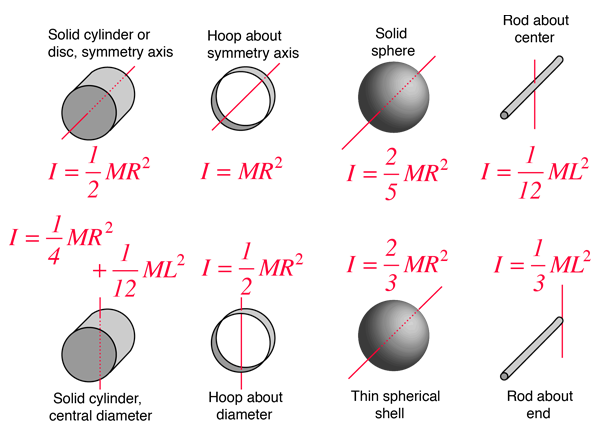# Rotational-Linear ParallelsMore comparisons between linear and angular motion
Index

Moment of inertia concepts

 HyperPhysics***** Mechanics ***** Rotation R Nave
Go Back

# Rotational-Linear ParallelsUsing a string through a tube, a mass is moved in a horizontal circle with angular velocity ω. If the string is pulled down so that the radius is half the original radius, then conservation of angular momentum dictates that the ball must have four times the angular velocity. This is because the product of moment of inertia and angular velocity must remain constant, and halving the radius reduces the moment of inertia by a factor of four.With the appropriate balance of force, a circular orbit can be produced by a force acting toward the center. Acting perpendicular to the velocity, it provides the necessary centripetal force to keep it in a circle. If a spinning wheel and axle is supported by one end of the axle, then the torque produced by the weight of the wheel and axle produces a torque that is perpendicular to the angular momentum of the wheel. This changes its direction but not its magnitude, causing the tip of the axle to trace out a circle. This is called precession, and is analogous to the orbit of a mass under a central force.
Index

Moment of inertia concepts

 HyperPhysics***** Mechanics ***** Rotation R Nave
Go Back

# Moment of Inertia

Moment of inertia is the name given to rotational inertia, the rotational analog of mass for linear motion. It appears in the relationships for the dynamics of rotational motion. The moment of inertia must be specified with respect to a chosen axis of rotation. For a point mass, the moment of inertia is just the mass times the square of perpendicular distance to the rotation axis, I = mr2. That point mass relationship becomes the basis for all other moments of inertia since any object can be built up from a collection of point masses.Common forms Examples General form Development for point mass
Index

Moment of inertia concepts

 HyperPhysics***** Mechanics ***** Rotation R Nave
Go Back

# Common Moments of InertiaIndex

Moment of inertia concepts

 HyperPhysics***** Mechanics ***** Rotation R Nave
Go Back

# Moment of Inertia Examples

Moment of inertia is defined with respect to a specific rotation axis. The moment of inertia of a point mass with respect to an axis is defined as the product of the mass times the distance from the axis squared. The moment of inertia of any extended object is built up from that basic definition. The general form of the moment of inertia involves an integral.Moments of inertia for common forms
 Where moment of inertia appears in physical quantities
Index

Moment of inertia concepts

 HyperPhysics***** Mechanics ***** Rotation R Nave
Go Back

# Moment of Inertia, General Form

Since the moment of inertia of an ordinary object involves a continuous distribution of mass at a continually varying distance from any rotation axis, the calculation of moments of inertia generally involves calculus, the discipline of mathematics which can handle such continuous variables. Since the moment of inertia of a point mass is defined bythen the moment of inertia contribution by an infinitesmal mass element dm has the same form. This kind of mass element is called a differential element of mass and its moment of inertia is given byNote that the differential element of moment of inertia dI must always be defined with respect to a specific rotation axis. The sum over all these mass elements is called an integral over the mass.Usually, the mass element dm will be expressed in terms of the geometry of the object, so that the integration can be carried out over the object as a whole (for example, over a long uniform rod).

Having called this a general form, it is probably appropriate to point out that it is a general form only for axes which may be called "principal axes", a term which includes all axes of symmetry of objects. The concept of moment of inertia for general objects about arbitrary axes is a much more complicated subject. The moment of inertia in such cases takes the form of a mathematical tensor quantity which requires nine components to completely define it.

 Examples of integration to get moment of inertia. Straight rod Cylinder Sphere
Index

Moment of inertia concepts

 HyperPhysics***** Mechanics ***** Rotation R Nave
Go Back